# MBNMAtime for Model-Based (Network) Meta-Analysis

## Introduction

This vignette demonstrates how to use MBNMAtime to perform meta-analysis of studies with multiple follow-up measurements in order to account for time-course relationships within a single or multiple treatment comparisons. This can either be performed by conducting Model-Based Network Meta-Analysis (MBNMA) to pool relative treatment effects, by conducting Model-Based Meta-Analysis (MBMA) to pool study arms, or a combination of the two methods within the same analysis.

Including all available follow-up measurements within a study makes use of all the available evidence in a way that maintains connectivity between treatments and explains how the response of the treatment changes over time, thus explaining heterogeneity and inconsistency that may be present in a standard Network Meta-Analysis (NMA). All models and analyses are implemented in a Bayesian framework, following an extension of the standard NMA methodology presented by (Lu and Ades 2004) and are run in JAGS (version 4.3.0 or later is required) (JAGS Computer Program 2017). For full details of time-course MBNMA methodology see Pedder et al. (2019).

This package has been developed alongside MBNMAdose, a package that allows users to perform dose-response MBNMA to allow for modelling of dose-response relationships between different agents within a network. However, they should not be loaded into R at the same time as there are a number of functions with shared names that perform similar tasks yet are specific to dealing with either time-course or dose-response data.

Within the vignette, some models have not been evaluated, or have been run with fewer iterations than would be necessary to achieve convergence and produce valid results in practice. This has been done to speed up computation and rendering of the vignette.

### Workflow within the package

Functions within MBNMAtime follow a clear pattern of use:

1. Load your data into the correct format using mb.network()
2. Analyse your data using mb.run(), or any of the available wrapper time-course functions
3. Test for consistency using functions like mb.nodesplit()
4. Examine model results using forest plots and treatment rankings
5. Use your model to predict responses using predict()

At each of these stages there are a number of informative graphs that can be generated to help understand the data and to make decisions regarding model fitting.

## Datasets Included in the Package

### Pain relief in osteoarthritis

osteopain is from a systematic review of treatments for pain in osteoarthritis, used previously in Pedder et al. (2019). The outcome is pain measured on a continuous scale, and aggregate data responses correspond to the mean WOMAC pain score at different follow-up times. The dataset includes 30 Randomised-Controlled Trials (RCTs), comparing 29 different treatments (including placebo). osteopain is a data frame in long format (one row per time point, arm and study), with the variables studyID, time, y, se, treatment and arm.

studyID time y se treatment arm treatname
Baerwald 2010 0 6.55 0.09 Pl_0 1 Placebo_0
Baerwald 2010 2 5.40 0.09 Pl_0 1 Placebo_0
Baerwald 2010 6 4.97 0.10 Pl_0 1 Placebo_0
Baerwald 2010 13 4.75 0.11 Pl_0 1 Placebo_0
Baerwald 2010 0 6.40 0.13 Na_1000 2 Naproxen_1000
Baerwald 2010 2 4.03 0.13 Na_1000 2 Naproxen_1000

### Alogliptin for lowering blood glucose concentration in type II diabetes

alog_pcfb is from a systematic review of Randomised-Controlled Trials (RCTs) comparing different doses of alogliptin with placebo (Langford et al. 2016). The systematic review was simply performed and was intended to provide data to illustrate a statistical methodology rather than for clinical inference. Alogliptin is a treatment aimed at reducing blood glucose concentration in type II diabetes. The outcome is continuous, and aggregate data responses correspond to the mean change in HbA1c from baseline to follow-up in studies of at least 12 weeks follow-up. The dataset includes 14 Randomised-Controlled Trials (RCTs), comparing 5 different doses of alogliptin with placebo (6 different treatments in total). alog_pcfb is a data frame in long format (one row per time point, arm and study), with the variables studyID, clinicaltrialGov_ID, agent, dose, treatment, time, y, se, and N.

studyID clinicaltrialGov_ID agent dose treatment time y se N
1 NCT01263470 alogliptin 0.00 placebo 2 0.00 0.02 75
1 NCT01263470 alogliptin 6.25 alog_6.25 2 -0.16 0.02 79
1 NCT01263470 alogliptin 12.50 alog_12.5 2 -0.17 0.02 84
1 NCT01263470 alogliptin 25.00 alog_25 2 -0.16 0.02 79
1 NCT01263470 alogliptin 50.00 alog_50 2 -0.15 0.02 79
1 NCT01263470 alogliptin 0.00 placebo 4 -0.01 0.04 75

### Body weight reduction in obesity patients

obesityBW_CFB is from a systematic review of pharmacological treatments for obesity. The outcome measured is change from baseline in body weight (kg) at different follow-up times. 35 RCTs are included that investigate 26 different treatments (16 agents/agent combinations compared at different doses). obesityBW_CFB is a dataset in long format (one row per time point, arm and study), with the variables studyID, time, y, se, N, treatment, arm, treatname, agent and agentclass.

agentclass is the class of a particular agent (e.g. Lipase inhibitor)

studyID time y se N treatment treatname agent class
27 Apfelbaum 1999 4.35 -1.00 0.39 78 plac placebo placebo Placebo
28 Apfelbaum 1999 4.35 -1.59 0.38 81 sibu_10MG sibutramine 10MG sibutramine SNRI
29 Apfelbaum 1999 8.70 -1.59 0.40 78 plac placebo placebo Placebo
30 Apfelbaum 1999 8.70 -3.01 0.39 81 sibu_10MG sibutramine 10MG sibutramine SNRI
31 Apfelbaum 1999 13.04 -2.25 0.41 78 plac placebo placebo Placebo
32 Apfelbaum 1999 13.04 -4.76 0.40 81 sibu_10MG sibutramine 10MG sibutramine SNRI

### Serum uric acid concentration in gout

goutSUA_CFB is from a systematic review of interventions for lowering Serum Uric Acid (SUA) concentration in patients with gout [not published previously]. The outcome is continuous, and aggregate data responses correspond to the mean change from baseline in SUA in mg/dL at different follow-up times. The dataset includes 28 RCTs, comparing 41 treatments (8 agents compared at different doses). goutSUA_CFB is a data frame in long format (one row per arm and study), with the variables studyID, time, y, se, treatment, arm, class and treatname.

studyID time y se treatment treatname class
1102 1 0.07 0.25 RDEA_100 RDEA594_100 RDEA
1102 1 0.02 0.18 RDEA_200 RDEA594_200 RDEA
1102 1 0.06 0.25 RDEA_400 RDEA594_400 RDEA
1102 2 -0.53 0.25 RDEA_100 RDEA594_100 RDEA
1102 2 -1.37 0.18 RDEA_200 RDEA594_200 RDEA
1102 2 -1.73 0.25 RDEA_400 RDEA594_400 RDEA

## Inspecting the data

Before embarking on an analysis, the first step is to have a look at the raw data. Two features (network connectivity and time-course relationship) are particularly important for MBNMA/MBMA. For this we want to get our dataset into the right format for the package. We can do this using mb.network(). This requires specifying the desired treatment to use for the network reference treatment.

# Using the pain dataset
network.pain <- mb.network(osteopain, reference = "Pl_0")
print(network.pain)
#> description :
#>  "Network"
#>
#> data.ab :
#> # A tibble: 417 x 10
#> # Groups:   studyID, time 
#>    studyID  time     y     se treatment   arm treatname fupcount  fups
#>    <fct>   <dbl> <dbl>  <dbl>     <dbl> <int> <fct>        <int> <int>
#>  1 Baerwa~     0  6.55 0.0861         1     1 Placebo_0        1     4
#>  2 Baerwa~     0  6.40 0.127         15     2 Naproxen~        1     4
#>  3 Baerwa~     0  6.62 0.0900        16     3 Naproxci~        1     4
#>  4 Baerwa~     2  5.40 0.0932         1     1 Placebo_0        2     4
#>  5 Baerwa~     2  4.03 0.133         15     2 Naproxen~        2     4
#>  6 Baerwa~     2  4.43 0.0926        16     3 Naproxci~        2     4
#>  7 Baerwa~     6  4.97 0.0997         1     1 Placebo_0        3     4
#>  8 Baerwa~     6  3.72 0.139         15     2 Naproxen~        3     4
#>  9 Baerwa~     6  4.08 0.0965        16     3 Naproxci~        3     4
#> 10 Baerwa~    13  4.75 0.109          1     1 Placebo_0        4     4
#> # ... with 407 more rows, and 1 more variable: narm <int>
#>
#> treatments :
#>   "Pl_0"    "Ce_100"  "Ce_200"  "Ce_400"  "Du_90"   "Et_10"   "Et_30"
#>   "Et_5"    "Et_60"   "Et_90"   "Lu_100"  "Lu_200"  "Lu_400"  "Lu_NA"
#>  "Na_1000" "Na_1500" "Na_250"  "Na_750"  "Ox_44"   "Ro_12"   "Ro_125"
#>  "Ro_25"   "Tr_100"  "Tr_200"  "Tr_300"  "Tr_400"  "Va_10"   "Va_20"
#>  "Va_5"

This takes a dataset with the columns:

• studyID Study identifiers
• time Numeric data indicating follow-up times
• y Numeric data indicating the mean response for a given observation
• se Numeric data indicating the standard error for a given observation
• treatment Treatment identifiers (can be numeric, factor or character)
• class An optional column indicating a particular class that may be shared by several treatments.
• N An optional column indicating the number of participants used to calculate the response at a given observation.

Additional columns can be included in the dataset. These will simply be added to the mb.network object, though will not affect the classification of the data.

mb.network then performs the following checks on the data: * The dataset has the required column names * There are no missing values * All standard errors (SE) are positive * Observations are made at the same time points in all arms of a study (i.e. the data are balanced) * Class labels are consistent within each treatment * Studies have at least two arms

And finally it converts the data into an object of class("mb.network"), which contains indices for study arms and follow-up measurements, and generates numeric values for treatments and classes. By convention, treatments are numbered alphabetically, though if the original data for treatments is provided as a factor then the factor codes will be used. This then contains all the necessary information for subsequent MBNMAtime functions.

### Network connectivity

Looking at how the evidence in the network is connected and identifying which studies compare which treatments helps to understand which treatment effects can be estimated and what information will be helping to inform those estimates. Network plots can be plotted which shows how treatments have been compared in head-to-head trials. Typically the thickness of connecting lines (“edges”) is proportional to the number of studies that make a particular comparison and the size of treatment nodes (“vertices”) is proportional to the total number of patients in the network who were randomised to a given treatment (provided N is included as a variable in the original dataset for mb.network()).

In MBNMAtime these plots are generated using igraph, and can be plotted by calling plot(). The generated plots are objects of class("igraph") meaning that, in addition to the options specified in plot(), various igraph functions can be used to make more detailed edits to them.

# Prepare data using the alogliptin dataset
network.alog <- mb.network(alog_pcfb, reference = "placebo")

# Plot network
plot(network.alog)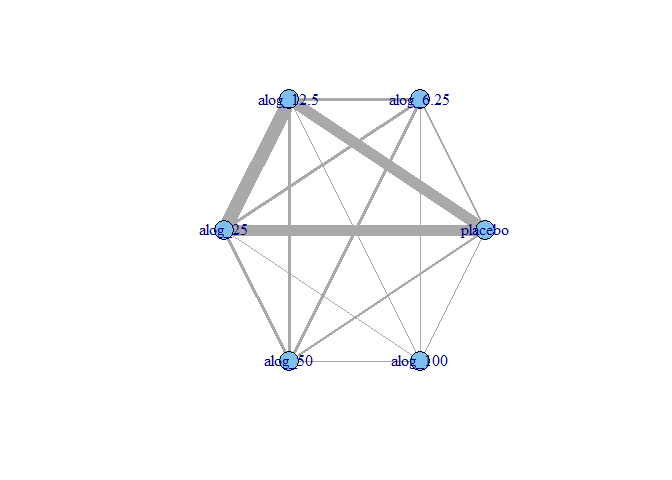Within these network plots, treatments are automatically aligned in a circle (as the default) and can be tidied by shifting the label distance away from the nodes.

# Draw network plot using the gout dataset
network.gout <- mb.network(goutSUA_CFB, reference = "Plac")
plot(network.gout, layout_in_circle = TRUE, label.distance = 5)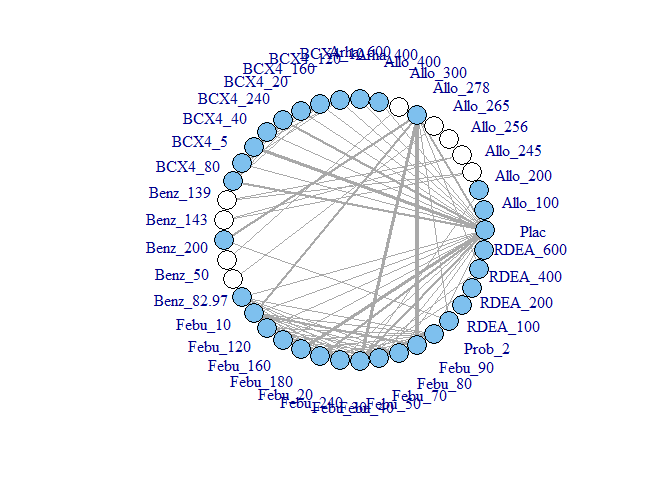This command returns a warning stating that some treatments are not connected to the network reference treatment through any pathway of head-to-head evidence. The nodes that are coloured white represent these treatments. This means that it will not be possible to estimate relative effects for these treatments versus the network reference treatment (or any treatments connected to it). Several options exist to allow for inclusion of these treatments in an analysis which we will discuss in more detail further on in the vignette, but one approach is to assume some sort of common effect among treatments within the same class/agent. We can generate a network plot at the class level to examine this more closely, and can see that the network is connected at the class level.

plot(network.gout, level = "class", remove.loops = TRUE, label.distance = 5)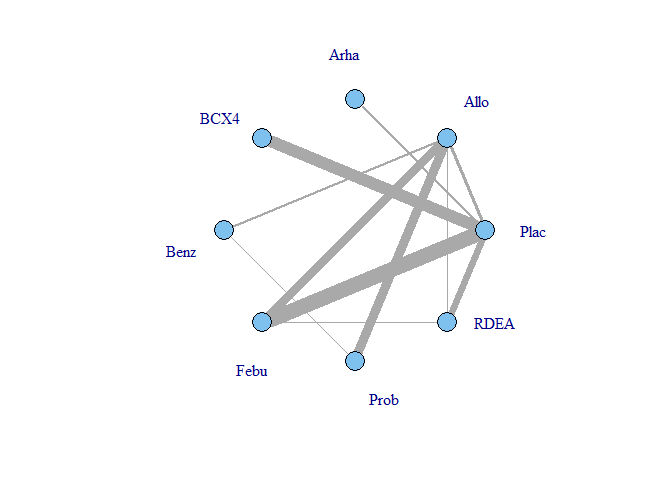It is also possible to plot a network at the treatment level but to colour the treatments by the class that they belong to.

plot(network.gout, level = "treatment", v.color = "class", label.distance = 5)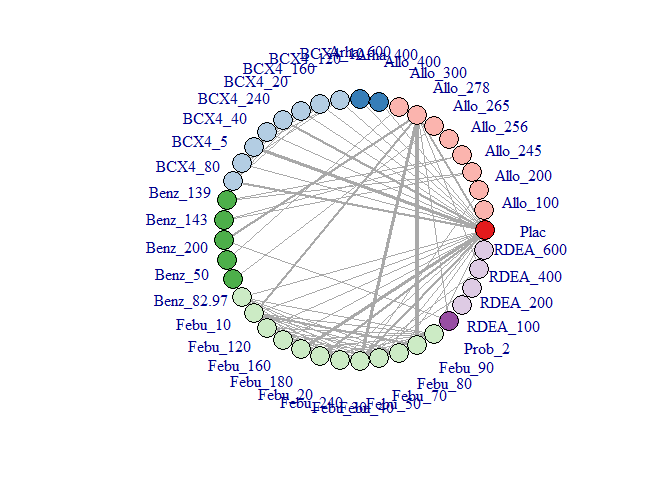For other analyses on the Gout dataset in this vignette we have taken the approach of combining several very similar doses to generate a network that is more completely connected:

network.gout <- mb.network(goutSUA_CFBcomb, reference="Plac")
plot(network.gout, label.distance = 5)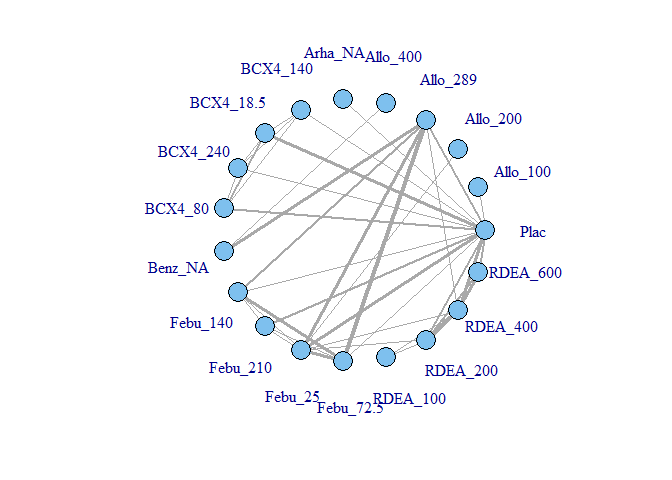### Examining the time-course relationship

In order to consider which functional forms may be appropriate for modelling the time-course relationship, it is important to look at the responses in each arm plotted over time. This can easily be done using the timeplot() function on an object of class("mb.network")

# Prepare data using the pain dataset
network.pain <- mb.network(osteopain, reference="Pl_0")

# Draw plot of raw study responses over time
timeplot(network.pain)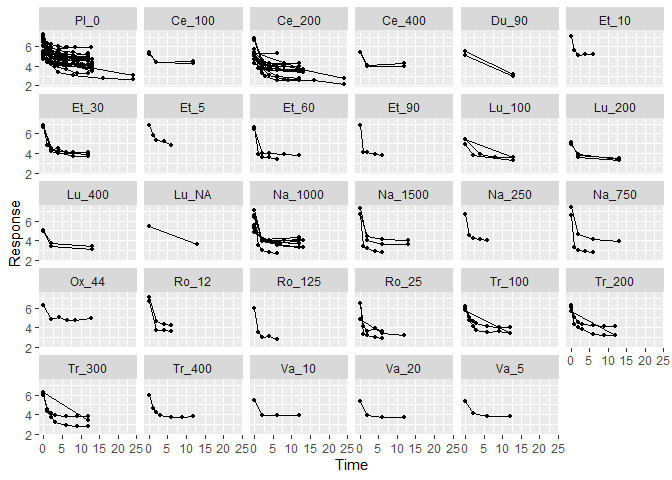As the mean response for all treatments shows a rapid reduction in pain score followed by a leveling out after 2-5 weeks, an exponential decay time-course function might be a reasonable fit for this dataset. More complex alternatives could be Emax models (with or without a Hill parameter), fractional polynomials or a linear piecewise function.

Responses can also be plotted grouped by some sort of class group rather than by treatment, which may give an indication of whether there are any similarities within a class:

# Draw plot of raw study responses over time grouped by agent class in the obesity dataset
network.obese <- mb.network(obesityBW_CFB)
timeplot(network.obese, level="class")
#> Absence of observations with time=0 in network - data assumed to be change from baseline:
#> plotting response=0 at time=0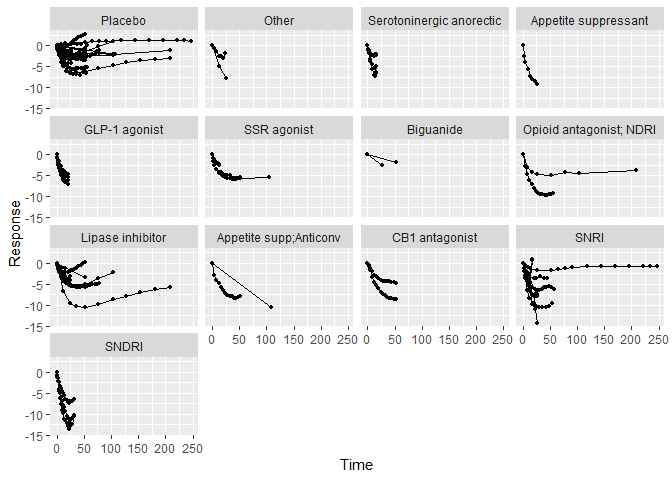In this example many of the profiles are quite different within the same class, which would suggest modelling class effects may be inappropriate.

## Analysis using mb.run()

MBNMA/MBMA is performed in the MBNMAtime by applying mb.run(). This can just as easily be performed on datasets with many different treatments (network meta-analysis) as it can on datasets comparing only two treatments (pairwise meta-analysis) - the syntax is the same.

An object or class("mb.network") must be provided as the data for mb.run(). The key arguments within mb.run() involve specifying the functional form used to model the time-course, and the time-course parameters that comprise that functional form.

#### Time-course functions

Several different functional forms are implemented within MBNMAtime, that allow a wide variety of parameterizations and time-course shapes. These are provided to the fun argument in mb.run():

• "linear"
• "quadratic"
• "exponential"
• "emax" - Emax without a Hill parameter
• "emax.hill" - Emax with a Hill parameter
• "fract.poly.first" - first-order fractional polynomial
• "fract.poly.second" - second-order fractional polynomial
• "piecelinear" - piecewise linear with a knot joining the two linear pieces
• "user" - A function that can be specified by the user within user.fun (see ?mb.run())

#### Time-course parameters

In mb.run() it is possible to specify up to four different time-course parameters, depending on the time-course function used. These are named beta.1, beta.2, beta.3 and beta.4, and their interpretation varies depending on the time-course function used (see ?mb.run()).

For simplification and interpretability, both in the way in which time-course parameters are defined and in how they are reported in the output, there are wrapper functions for mb.run() for each of the provided time-course functions. For example, mb.emax() is equivalent to mb.run(fun="emax"), but with a different naming of time-course parameters (emax instead of beta.1 and et50 instead of beta.2) . A number of these wrapper functions will be shown in other examples in this vignette.

Time-course parameters can be assigned several different specifications, which define the key parameters estimated by the model. These are defined within a list that contains two named elements, pool and method.

pool is used to define the approach used for the pooling of a given time-course parameter and can take any of the following values:

• "rel" indicates that relative effects should be pooled for this time-course parameter. This preserves randomisation within included studies, are likely to vary less between studies (only due to effect modification), and allow for testing of consistency between direct and indirect evidence. Pooling follows the general approach for Network Meta-Analysis proposed by Lu and Ades (2004).
• "arm" indicates that study arms should be pooled within each treatment in the network for this time-course parameter. This allows estimation of absolute time-course parameter values, but makes stronger assumptions regarding similarity of studies.
• "const" indicates that study arms should be pooled across the whole network for this time-course parameter independently of assigned treatment. This implies using a single value across the network for this time-course parameter, and may therefore be making very strong assumptions of similarity.

method is used to define the model used for meta-analysis for a given time-course parameter and can take any of the following values:

• "common" implies that all studies estimate the same true effect (akin to a “fixed effects” meta-analysis)
• "random" implies that all studies estimate a separate true effect, but that each of these true effects vary randomly around a true mean effect. This approach allows for modelling of between-study heterogeneity.
• numeric() Assigned a numeric value - this can only be used if pool="const". It indicates that this time-course parameter should not be estimated from the data but should be assigned the numeric value determined by the user. This can be useful for fixing specific time-course parameters (e.g. Hill parameters in Emax functions or knot location in piecewise functions).

Specifying pooling relative effects (pool="rel") on all time-course parameters would imply performing a contrast-based MBNMA, whereas specifying pooling arm effects (pool="arm") on all of them would imply performing an arm-based MBMA. There has been substantial discussion in the literature regarding the strengths and limitations of both these approaches (Dias and Ades 2016; Hong et al. 2016; Karahalios et al. 2017).

### Output

mb.run() returns an object of class(c("mbnma", "rjags")). summary() provides posterior medians and 95% credible intervals for different parameters in the model, with some explanation regarding the way in which the model has been defined. print() can also be used to give summary statistics of the posterior distributions for monitored nodes in the JAGS model. Estimates are automatically reported for parameters of interest depending on the model specification (unless otherwise specified in parameters.to.save)

Nodes that are automatically monitored (if present in the model) have the following interpretation. They will have an additional suffix that relates to the name/number of the time-course parameter to which they correspond (e.g. d.et50 or beta.1):

• d The pooled relative effect for a given treatment compared to the network reference treatment for a particular time-course parameter, reported if pool="rel"
• sd.d The between-study standard deviation (SD) or heterogeneity for relative effects, reported if pool="rel" and method="random"
• D The class effect for a given class relative to the network reference class for a particular time-course parameter. This will be reported if class effects are applied to a parameter for which method="rel".
• sd.D The standard deviation for the pooled relative effects of treatments within a given class from a model with a random class effect.
• beta If pool="const" then only a single node will be present in the output, which corresponds to the absolute value of a particular time-course parameter across the network, If pool="arm" then for the relevant time-course parameter there will be one node for each treatment, which represents the absolute value of the time-course parameter for each treatment
• sd.beta Reported if method="random" and pool is either "const" or "arm". If pool="const" this represents the between-study SD for the absolute value of a particular time-course parameter exchangeable across the network. If pool="arm" this represents the between-study SD for the absolute value of a particular time-course parameter exchangeable by treatment
• BETA The class effect for a given class for a particular time-course parameter, reported as an absolute value. This will be reported if class effects are applied to a parameter for which method="arm".
• sd.BETA The standard deviation for the pooled absolute effects of treatments within a given class from a model with a random class effect.
• rho The correlation coefficient for correlation between time-points. Its interpretation will differ depending on the covariance structure used
• totresdev The residual deviance of the model
• deviance The deviance of the model

Model fit statistics for pD (effective number of parameters) and DIC (Deviance Information Criterion) are also reported, with an explanation as to how they have been calculated.

#### Examples

An example MBNMA of the pain dataset using an exponential time-course function and fixed treatment effects that pool relative effects and assumes consistency between direct and indirect evidence can be performed as follows:

# Run a linear time-course MBNMA
mbnma <- mb.run(network.pain, fun="linear",
beta.1=list(pool="rel", method="common"))
#> module glm loaded
summary(mbnma)
#> ========================================
#> Time-course MBNMA
#> ========================================
#>
#> Time-course function: linear
#> Data modelled with intercept
#>
#>
#> #### beta.1 time-course parameter pooling ####
#> Pooling: relative effects
#> Method: common (fixed) effects
#>
#> Estimated from the data:
#>
#> Parameter        Median (95%CrI)
#> ---------------------------------
#> d.1   Network reference
#> d.1   -0.0436 (-0.0628, -0.0243)
#> d.1   -0.0601 (-0.0659, -0.0543)
#> d.1   -0.0729 (-0.0902, -0.0553)
#> d.1   -0.0427 (-0.0688, -0.0177)
#> d.1   0.102 (0.0492, 0.156)
#> d.1   -0.0975 (-0.11, -0.085)
#> d.1   0.0724 (0.0208, 0.126)
#> d.1   -0.158 (-0.18, -0.136)
#> d.1  -0.206 (-0.26, -0.152)
#> d.1  -0.0601 (-0.0692, -0.0512)
#> d.1  -0.0561 (-0.0662, -0.0461)
#> d.1  -0.0694 (-0.0812, -0.0578)
#> d.1  -0.0659 (-0.0832, -0.0494)
#> d.1  -0.0979 (-0.108, -0.0881)
#> d.1  -0.0929 (-0.107, -0.079)
#> d.1  0.156 (0.105, 0.209)
#> d.1  -0.063 (-0.0822, -0.0446)
#> d.1  -0.121 (-0.169, -0.0748)
#> d.1  -0.076 (-0.109, -0.0411)
#> d.1  -0.39 (-0.463, -0.321)
#> d.1  -0.108 (-0.139, -0.0794)
#> d.1  -0.0165 (-0.032, -0.000988)
#> d.1  -0.0257 (-0.0411, -0.0107)
#> d.1  -0.0617 (-0.0767, -0.047)
#> d.1  -0.0751 (-0.0958, -0.0529)
#> d.1  -0.0536 (-0.078, -0.0289)
#> d.1  -0.0788 (-0.103, -0.0542)
#> d.1  -0.0648 (-0.0899, -0.0401)
#>
#>
#>
#>
#> #### Model Fit Statistics ####
#>
#> Effective number of parameters:
#> pD (pV) calculated using the rule, pD = var(deviance)/2 = 85
#> Deviance = 8893
#> Residual deviance = 9773
#> Deviance Information Criterion (DIC) = 8978
# An alternative would be to use a linear wrapper for mb.run() which would give the same result
mb.linear(network.pain,
slope=list(pool="rel", method="common"))

In this case the d.1 (or d.slope when using the wrapper function) parameters correspond to the relative effects for each treatment versus the network reference treatment for the time-course parameter beta.1, which corresponds to the linear slope/gradient.

Instead of pooling relative effects, a different approach could be to use MBMA to pool absolute time-course parameter affects within the same treatment. The following is an example MBMA of the gout dataset which uses an Emax time-course function, with random effects for pooling absolute Emax (beta.1) within different treatments and modelling a single common parameter for ET50 (beta.2) estimated across all treatments within the network. As the gout data is reported as change from baseline, we do not include an intercept in the model, forcing the response to be zero at time = 0.

# Run an emax time-course MBNMA pooling absolute effects
mbnma <- mb.run(network.gout, fun="emax",
beta.1=list(pool="arm", method="random"),
beta.2=list(pool="const", method="common"),
intercept=FALSE)
summary(mbnma)
#> ========================================
#> Time-course MBNMA
#> ========================================
#>
#> Time-course function: emax
#> Data modelled without intercept (change from baseline data assumed)
#>
#>
#> #### beta.1 time-course parameter pooling ####
#> Pooling: arm-based
#> Method: random effects
#>
#> Estimated from the data:
#>
#> Parameter        Median (95%CrI)
#> ---------------------------------
#> beta.1    -2.27 (-0.464, -4.94)
#> beta.1    0.02 (-0.464, 0.503)
#> beta.1    -0.569 (-2.27, 1.07)
#> beta.1    -3.21 (-4.94, -1.57)
#> beta.1    -3.25 (-3.78, -2.73)
#> beta.1    -3.4 (-5.14, -1.71)
#> beta.1    -1.68 (-3.39, 0.00645)
#> beta.1    -3.29 (-4.92, -1.57)
#> beta.1    -1.28 (-2.22, -0.323)
#> beta.1    -4.21 (-5.9, -2.56)
#> beta.1   -2.98 (-4.15, -1.73)
#> beta.1   -4.64 (-5.46, -3.82)
#> beta.1   -4.63 (-5.57, -3.63)
#> beta.1   -5.28 (-6.52, -4.05)
#> beta.1   -3.18 (-3.77, -2.56)
#> beta.1   -4.19 (-4.93, -3.42)
#> beta.1   -0.598 (-2.31, 1.08)
#> beta.1   -1.77 (-2.61, -0.945)
#> beta.1   -2.74 (-3.41, -2.04)
#> beta.1   -3.58 (-4.53, -2.58)
#>
#> # Between-study SD
#>
#> Parameter        Median (95%CrI)
#> ---------------------------------
#> sd.beta.1        0.845 (0.693, 1.05)
#>
#>
#>
#> #### beta.2 time-course parameter pooling ####
#> Parameter modelled on exponential scale to ensure it takes positive values on the natural scale
#> Pooling: constant (single parameter constant across all studies and treatments within the network)
#> Method: common (fixed) effects
#>
#> Estimated from the data:
#>
#> Parameter        Median (95%CrI)
#> ---------------------------------
#> beta.2       -74.6 (-230, -11.3)
#>
#>
#>
#>
#> #### Model Fit Statistics ####
#>
#> Effective number of parameters:
#> pD (pV) calculated using the rule, pD = var(deviance)/2 = 68
#> Deviance = 2127
#> Residual deviance = 2821
#> Deviance Information Criterion (DIC) = 2195
# An alternative would be to use an emax wrapper for mb.run() which would give the same result
mb.emax(network.gout,
emax=list(pool="arm", method="random"),
et50=list(pool="const", method="common"),
intercept=FALSE)

In this case the beta.1 parameters in the output correspond to the absolute effect for each treatment for the time-course parameter beta.1, which corresponds to Emax. beta.2 corresponds to the overall absolute effect for the time-course parameter beta.2, which corresponds to ET50. sd.beta.1 is the between-study SD for the absolute effect of beta.1.

Finally, we could perform an analysis that combines both relative and absolute pooling approaches on different time-course parameters. For example an analysis of the pain dataset that models a piecewise linear time-course with fixed pooling of relative treatment effects on the slope of the first linear piece (beta.1) and fixed pooling of absolute treatment effects on the slope of the second linear piece (beta.2), with a knot (beta.3) at 1 week follow-up defined in the model (rather than estimated from the data.

# Run a piecewise linear time-course MBNMA
mbnma <- mb.run(network.pain, fun="piecelinear",
beta.1=list(pool="rel", method="common"),
beta.2=list(pool="arm", method="common"),
beta.3=list(pool="const", method=1))
summary(mbnma)
#> ========================================
#> Time-course MBNMA
#> ========================================
#>
#> Time-course function: piecelinear
#> Data modelled with intercept
#>
#>
#> #### beta.1 time-course parameter pooling ####
#> Pooling: relative effects
#> Method: common (fixed) effects
#>
#> Estimated from the data:
#>
#> Parameter        Median (95%CrI)
#> ---------------------------------
#> d.1   Network reference
#> d.1   -0.91 (-1.17, -0.674)
#> d.1   -0.922 (-1.01, -0.838)
#> d.1   -1.24 (-1.47, -1)
#> d.1   0.569 (-59.5, 62.9)
#> d.1   -0.263 (-0.611, 0.0925)
#> d.1   -1.06 (-1.2, -0.907)
#> d.1   0.18 (-0.151, 0.529)
#> d.1   -1.78 (-1.98, -1.58)
#> d.1  -1.49 (-1.83, -1.15)
#> d.1  -0.815 (-0.977, -0.657)
#> d.1  -0.847 (-0.98, -0.715)
#> d.1  -1.06 (-1.21, -0.903)
#> d.1  -0.0559 (-60.5, 64.5)
#> d.1  -1.33 (-1.44, -1.21)
#> d.1  -1.08 (-1.23, -0.929)
#> d.1  0.248 (-0.096, 0.617)
#> d.1  -0.856 (-1.04, -0.659)
#> d.1  -1.44 (-2.03, -0.849)
#> d.1  -0.707 (-0.996, -0.393)
#> d.1  -1.85 (-2.28, -1.4)
#> d.1  -1.18 (-1.43, -0.936)
#> d.1  0.0202 (-0.14, 0.175)
#> d.1  -0.109 (-0.263, 0.0462)
#> d.1  -0.494 (-0.652, -0.333)
#> d.1  -0.585 (-0.794, -0.381)
#> d.1  -0.991 (-1.28, -0.711)
#> d.1  -1.04 (-1.33, -0.755)
#> d.1  -0.824 (-1.13, -0.54)
#>
#>
#>
#> #### beta.2 time-course parameter pooling ####
#> Pooling: arm-based
#> Method: common (fixed) effects
#>
#> Estimated from the data:
#>
#> Parameter        Median (95%CrI)
#> ---------------------------------
#> beta.2    -0.0237 (-0.0638, -0.04)
#> beta.2    -0.057 (-0.0638, -0.0501)
#> beta.2    0.0032 (-0.0237, 0.0311)
#> beta.2    -0.0329 (-0.04, -0.0256)
#> beta.2    0.0063 (-0.0184, 0.0319)
#> beta.2    -0.145 (-4.94, 4.47)
#> beta.2    -0.048 (-0.134, 0.0396)
#> beta.2    -0.0483 (-0.0652, -0.0303)
#> beta.2    -0.179 (-0.264, -0.0988)
#> beta.2    -0.00353 (-0.0306, 0.0248)
#> beta.2   -0.0696 (-0.154, 0.0141)
#> beta.2   -0.0427 (-0.0576, -0.0268)
#> beta.2   -0.0344 (-0.0461, -0.0219)
#> beta.2   -0.0272 (-0.0414, -0.0127)
#> beta.2   -0.111 (-5.07, 4.54)
#> beta.2   -0.0104 (-0.0222, 0.00181)
#> beta.2   -0.0388 (-0.0569, -0.0213)
#> beta.2   -0.0933 (-0.187, -0.00368)
#> beta.2   -0.0387 (-0.0646, -0.0144)
#> beta.2   0.00162 (-0.0678, 0.0676)
#> beta.2   -0.0703 (-0.14, -0.00547)
#> beta.2   -0.102 (-0.209, 0.00809)
#> beta.2   -0.0248 (-0.0705, 0.0207)
#> beta.2   -0.0924 (-0.112, -0.0727)
#> beta.2   -0.0865 (-0.107, -0.0669)
#> beta.2   -0.0773 (-0.0973, -0.058)
#> beta.2   -0.0669 (-0.0955, -0.0396)
#> beta.2   0.000458 (-0.0361, 0.0347)
#> beta.2   -0.019 (-0.0551, 0.0145)
#> beta.2   -0.0295 (-0.0641, 0.00915)
#>
#>
#>
#> #### beta.3 time-course parameter pooling ####
#> Pooling: constant (single parameter constant across all studies and treatments within the network)
#> Method: none
#>
#> Assigned a numeric value: 1
#>
#>
#>
#>
#>
#> #### Model Fit Statistics ####
#>
#> Effective number of parameters:
#> pD (pV) calculated using the rule, pD = var(deviance)/2 = 112
#> Deviance = -11
#> Residual deviance = 869
#> Deviance Information Criterion (DIC) = 101
# An alternative would be to use a piecewise linear wrapper for mb.run() which would give the same result
mb.piecelinear(network.pain,
slope.1=list(pool="rel", method="common"),
slope.2=list(pool="arm", method="common"),
knot=list(pool="const", method=1),
n.iter=5000)

This automatically monitors d.1, the relative effect for each treatment versus the network reference treatment of the slope of the first linear piece, and beta.1, the absolute value of the slope of the second linear piece for each treatment. beta.3 is not shown in the output as this is defined in the model as taking the value of 1 and has not been estimated from the data.

### Additional model specification with mb.run()

#### Correlation between time points

Correlation between time points can easily be modelled using mb.run(), though this requires some additional considerations . The simplest approach is to set rho = "estimate" in mb.run(), which will estimate rho from the data, and then define a covariance structure using covar. A simple compound symmetry ("CS") covariance structure can be used, which assumes the same correlation between responses at any two time points. Alternatively, an autoregressive AR1 structure ("AR1"), allows for responses at closer time points to be more strongly correlated.

As with beta time-course parameters in the model, we can also assign rho a numeric value if we do not want to estimate it within the model. For example this could be estimated from external data, or one might wish to run a deterministic sensitivity analysis with different value of rho.

As these models account for correlation by using a multivariate likelihood, the analysis is much slower to run, and it is therefore not shown for the purposes of this vignette.

# Run an emax time-course MBNMA that accounts for correlation between time points using a wrapper for mb.run()
mbnma <- mb.emax(network.pain,
emax=list(pool="rel", method="common"),
et50=list(pool="const", method="common"),
rho="estimate", covar="CS")

Since rho is estimated from the data in this model, we will also have summary statistics for it in the output.

It is important to note that the covariance matrix must be positive semi-definite. This may mean that in order to satisfy this requirement for particular covariance matrix structures, the values that rho can take are limited. In some instances the default prior distribution for rho (dunif(-1,1)) can lead to an error in the evaluation of the multivariate likelihood, in which case it may be necessary to restrict the prior distribution. This can be done using the priors argument (see ?mb.run() and the section below on priors).

### Class effects

Shared effects between treatments within the network can be modelled using class effects. This requires assuming that different treatments have some sort of common class effect, perhaps due to different (yet similar) doses of the same agent or different treatments with a similar mechanism of action. One advantage of this is that class effects can be used to connect relative effects between treatments in a network that are connected at the class level but that might otherwise be disconnected at the treatment level. However, it is important to ensure that such an effect is clinically justifiable, as making such a strong assumption when there is not true similarity may introduce heterogeneity/inconsistency.

Class effects can only be applied to time-course parameters which vary by treatment (either pool="rel" or pool="arm").

In mb.run() class effects are supplied as a list, in which each element is named following the name of the corresponding time-course parameter as defined in the function. The names will therefore differ when using wrapper functions for mb.run(). The class effect for each time-course parameter can be either "common", in which the effects for each treatment within the same class are constrained to a common class effect, or "random", in which the effects for each treatment within the same class are assumed to be randomly distributed around a shared class mean.

# Run an emax time-course MBNMA with a random class effects on beta.1 (Emax parameters)
# Additional iterations run to ensure MCMC chain convergence
mbnma <- mb.run(network.gout, fun="emax",
beta.1=list(pool="rel", method="random"),
beta.2=list(pool="const", method="common"),
intercept=FALSE, n.iter=20000,
class.effect=list(beta.1="random"))
#> ET50 parameters (beta.2) are on exponential scale to ensure they take positive values on the natural scale
summary(mbnma)
#> ========================================
#> Time-course MBNMA
#> ========================================
#>
#> Time-course function: emax
#> Data modelled without intercept (change from baseline data assumed)
#>
#>
#> #### beta.1 time-course parameter pooling ####
#> Pooling: relative effects
#> Method: random effects
#>
#> Estimated from the data:
#>
#> --- CLASS EFFECTS MODELLED (results shown in separate section below) ---
#>
#> # Between-study SD
#>
#> Parameter        Median (95%CrI)
#> ---------------------------------
#> sd.1     0.776 (0.586, 1.07)
#>
#>
#>
#> #### beta.2 time-course parameter pooling ####
#> Parameter modelled on exponential scale to ensure it takes positive values on the natural scale
#> Pooling: constant (single parameter constant across all studies and treatments within the network)
#> Method: common (fixed) effects
#>
#> Estimated from the data:
#>
#> Parameter        Median (95%CrI)
#> ---------------------------------
#> beta.2       -74.3 (-224, -11.5)
#>
#>
#>
#>
#> #### Class effects ####
#>
#> Class effect on beta.1: random
#>
#> Parameter    Median (95%CrI)
#> ---------------------------------
#> D.1   Network reference
#> D.1   -1.98 (-3.26, -0.566)
#> D.1   -2.1 (-4.83, 0.67)
#> D.1   -2.45 (-3.83, -1.09)
#> D.1   -3.56 (-5.98, -1.27)
#> D.1   -3.75 (-5.12, -2.55)
#> D.1   -2.25 (-3.53, -0.908)
#>
#> # Within-class SD
#>
#> Parameter    Median (95%CrI)
#> ---------------------------------
#> sd.D.1       0.983 (0.548, 1.73)
#>
#>
#> #### Model Fit Statistics ####
#>
#> Effective number of parameters:
#> pD (pV) calculated using the rule, pD = var(deviance)/2 = 68
#> Deviance = 2127
#> Residual deviance = 2821
#> Deviance Information Criterion (DIC) = 2195

Mean class effects are given in the output as D.1 parameters. These can be interpreted as the relative effect of each class versus the network reference class, for Emax parameters (beta.1). Note the number of D.1 parameters is therefore equal to the number of classes defined in the dataset.

As we have specified that the class effects are "random", each treatment effect for Emax (beta.1) is randomly distributed around its class mean with SD given in the output as sd.D.1.

Alternatively if we apply class effects to a time-course parameter pooled using "arm" then the outputs are in terms of absolute class effects (BETA and sd.BETA).

Several additional arguments can be given to mb.run() that require further explanation.

#### Absolute or change from baseline data

intercept can be used to specify whether or not the time-course function should include baseline (alpha) parameters. These are nuisance parameters in the model, and are not important to monitor, but if we are using change from baseline (CFB) data then we can set intercept = FALSE to define that the baseline will be zero (no change from baseline at time = 0), which reduces the number of parameters that need to be estimated within the model. We have done this for analyses of the gout, obesity and alogliptin datasets.

#### User-specified time-course function

If users want to write their own time-course function rather than using one of the ones specified in mb.run() they can do this by specifying fun = "user" in the arguments. A string can then be provided to user.fun, which specifies a new time course in terms of alpha, beta and time parameters. This allows a huge amount of additional flexibility when defining the time-course function.

The string assigned to user.fun needs to fulfill a few criteria to be valid: * alpha must be specified within the function. If modelling change from baseline data alpha still needs to be included, but users can set intercept = FALSE (see above). * At least one beta time-course parameter must be specified, up to a maximum of four. These are always named beta.1, beta.2, beta.3 and beta.4 (even if only one is specified), and must be included sequentially (i.e. don’t include beta.3 if beta.1 is not included) * time must be included in the function. It can be included as multiple instances as is necessary * Indices used by JAGS should not be added to the function (e.g. use alpha rather than alpha[i]) * Any mathematical/logical operators that can be implemented in JAGS can be added to the function

An example user-specified time-course function could be the following:

"alpha + (beta.1*time) * ((time/24)^beta.2)"

#### Priors

Default vague priors for the model are as follows:

\begin{aligned} &d_{\phi,t} \sim N(0,10000)\\ &beta_{\phi,t} \sim N(0,10000)\\ &D_{\phi,c} \sim N(0,1000)\\ &BETA_{\phi,c} \sim N(0,1000)\\ &rho \sim U(-1,1)\\ &\sigma_{\phi,d} \sim N(0,400) \text{ limited to } x \in [0,\infty]\\ &\sigma_{beta_\phi} \sim N(0,400) \text{ limited to } x \in [0,\infty]\\ &\sigma_{D_\phi} \sim N(0,400) \text{ limited to } x \in [0,\infty]\\ &\sigma_{BETA_\phi} \sim N(0,400) \text{ limited to } x \in [0,\infty]\\ \end{aligned}

…where $$\phi$$ is an index for the time-course parameter, $$t$$ a treatment index and $$c$$ a class index.

Users may wish to change these, perhaps in order to use more/less informative priors, but also because the default prior distributions in some models may lead to errors when compiling/updating models.

This can be more likely for certain types of models. For example for multivariate models, the covariance matrix must be positive semi-definite, and some values of the correlation coefficient, rho, will not fulfil this. Another example might be when using values that might generate results that are too extreme for JAGS to computer, such as for time-course parameters that are powers (e.g. Emax functions with a Hill parameter or power parameters in fractional polynomials).

If the model fails during compilation/updating (i.e. due to a problem in JAGS), mb.run() will generate an error and return a list of arguments that mb.run() used to generate the model. Within this (as within a model that has run successfully), the priors used by the model (in JAGS syntax) are stored within "model.arg".

In this way a model can first be run with vague priors and then rerun with different priors, perhaps to allow successful computation, perhaps to provide more informative priors, or perhaps to run a sensitivity analysis with different priors. Increasing the precision of prior distributions only a little can also often improve convergence considerably.

To change priors within a model, a list of replacements can be provided to priors in mb.run(). The name of each element is the name of the parameter to change (without indices) and the value of the element is the JAGS distribution to use for the prior. This can include censoring or truncation if desired. Only the priors to be changed need to be specified - priors for parameters that aren’t specified will take default values.

For example, previously in the vignette we were showing how correlation between time points could be modelled using a multivariate likelihood. However, if we use an autoregressive AR1 covariance structure with the default prior for rho this can result in a covariance matrix that is non-positive semidefinite, causing an error:

# Run an MBNMA that accounts for correlation between time points using AR1 covariance structure
mbnma <- mb.emax(network.pain,
emax=list(pool="rel", method="common"),
et50=list(pool="const", method="common"),
rho="estimate", covar="AR1")

print(mbnma$model.arg$priors)
#> ET50 parameters (beta.2) are on exponential scale to ensure they take positive values on the natural scale
#> Error in update.jags(object, n.iter, ...): RUNTIME ERROR:
#> unable to calculate eigenvalues in dsyev

We can solve this issue by defining a new prior within the model for rho that is limited to take only positive values:

# Define prior for rho which permits evaluation
new.priors <- list(
"rho" = "dunif(0, 1)"
)

# Run an MBNMA model with new priors
mbnma <- mb.emax(network.pain,
emax=list(pool="rel", method="common"),
et50=list(pool="const", method="common"),
rho="estimate", covar="AR1",
priors=new.priors)

#### pD (effective number of parameters)

The default value for pd in mb.run() is "pv", which uses the value automatically calculated in the R2jags package as pv = var(deviance)/2. Whilst this is easy to calculate, it is only an approximation to the effective number of parameters, and may be numerically unstable (Gelman et al. 2003).

A commonly-used approach for calculating pD is the plug-in method (pd="plugin") (Spiegelhalter et al. 2002). However, this can sometimes result in negative non-sensical values due to skewed posterior distributions for deviance contributions that can arise when fitting non-linear models.

Another exact approach that is more reliable than the plug-in method when modelling non-linear effects is using the Kullback-Leibler divergence (pd="pd.kl") (Plummer 2008). The disadvantage of this approach is that it requires running additional MCMC iterations, so can be slightly slower to calculate.

Finally, pD can also be calculated using an optimism adjustment (pd="popt") which allows for calculation of the penalized expected deviance (Plummer 2008). This adjustment allows for the fact that data used to estimate the model is the same as that used to assess its parsimony. It also requires running additional MCMC iterations.

#### Correlation between time-course parameters

mb.run() automatically models correlation between relative effect time-course parameters, as these are typically correlated and allows information on each parameter to help inform the other(s). The correlation is modelled using a vague Wishart prior, but this can be made more informative by indicating the relative magnitude of scales of the parameters that are modelled using relative effects.

var.scale can be used for this - it takes a numeric vector the same length as the number of relative effect time-course parameters (those modelled using pool="rel"), and the relative magnitude of the numbers indicates the relative magnitude of the scales. Each element of var.scale corresponds to the relevant time-course parameter (i.e. var.scale will correspond to the first time-course parameter modelled using relative effects)

For example, with the osteoarthritis dataset we might expect that for a piecewise linear time-course function, the parameter values (in this model the relative different in gradient vs placebo) for the first linear piece might be 10 times larger than for the second linear piece:

# Define relative magnitudes of slope.1 and slope.2
rel.size <- c(10,1)

mbnma <- mb.piecelinear(network.pain,
slope.1=list(pool="rel", method="random"),
slope.2=list(pool="rel", method="common"),
knot=list(pool="const", method=1),
var.scale = rel.size)

#### Arguments to be sent to JAGS

In addition to the arguments specific to mb.run() it is also possible to use any arguments to be sent to R2jags::jags(). Most of these are likely to relate to improving the performance of MCMC simulations in JAGS. Some of the key arguments that may be of interest are:

• n.chains The number of Markov chains to run (default is 3)
• n.iter The total number of iterations per MCMC chain
• n.burnin The number of iterations that are discarded to ensure iterations are only saved once chains have converged
• n.thin The thinning rate which ensures that results are only saved for 1 in every n.thin iterations per chain. This can be increased to reduce autocorrelation

## Post-Estimation

### Deviance plots

To assess how well a model fits the data, it can be useful to look at a plot of the contributions of each data point to the total deviance or residual deviance. This can be done using devplot(). As individual deviance contributions are not automatically monitored in the model, this might require the model to be run for additional iterations.

Results can be plotted either as a scatter plot (plot.type="scatter") or a series of boxplots (plot.type="box").

# Run a first-order fractional polynomial time-course MBNMA
mbnma <- mb.fract.first(network.pain,
slope=list(pool="rel", method="random"),
power=list(pool="const", method="common")
)

# Plot a scatter plot of residual deviance contributions (the default)
devplot(mbnma, n.iter=1000)
#> resdev not monitored in mbnma$parameters.to.save. #> additional iterations will be run in order to obtain results for resdev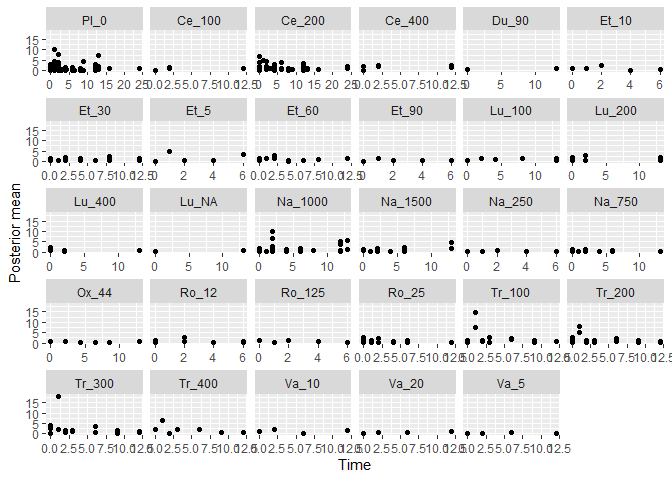# Plot boxplots of deviance contributions devplot(mbnma, dev.type = "dev", plot.type = "box", n.iter=1000) #> dev not monitored in mbnma$parameters.to.save.
#> additional iterations will be run in order to obtain results for dev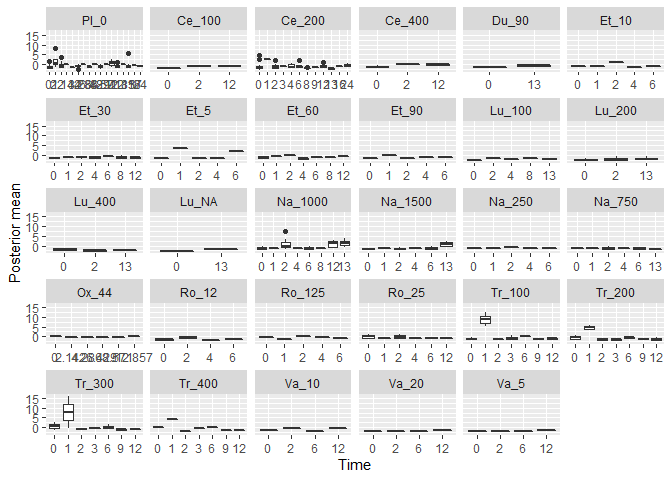From these plots we can see that whilst the model fit is good at later time points, it underestimates responses at earlier time points and can hugely overestimate that in the middle of the time-course function.

A function that appropriately captures the time-course shape should not show a flat shape of deviance contributions (i.e. contributions should be similar across all time points).

If saved to an object, the output of devplot() contains the results for individual deviance contributions, and this can be used to identify any extreme outliers.

### Fitted values

Another approach for assessing model fit can be to plot the fitted values, using fitplot(). As with devplot(), this may require running additional model iterations to monitor theta.

# Plot fitted and observed values with treatment labels
fitplot(mbnma, n.iter=1000)
#> theta not monitored in mbnma$parameters.to.save. #> additional iterations will be run in order to obtain results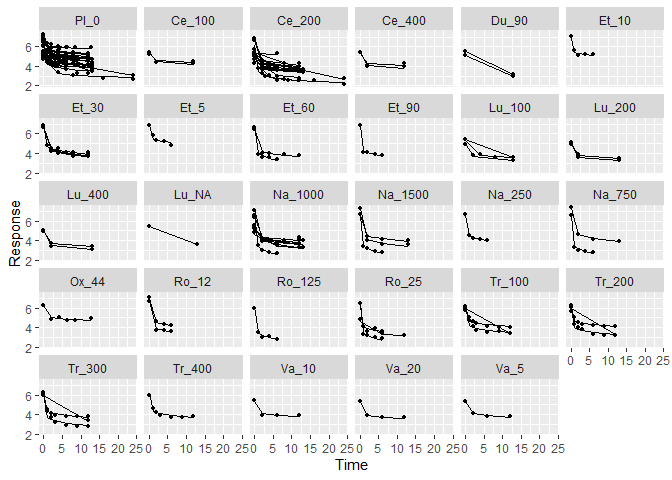Fitted values are plotted as connecting lines and observed values in the original dataset are plotted as points. These plots can be used to identify if the model fits the data well for different treatments and at different parts of the time-course. ### Forest plots Forest plots can be easily generated from MBNMA models using the plot() method on an "mbnma" object. By default this will plot a separate panel for each time-course parameter in the model. Forest plots can only be generated for parameters which vary by treatment/class. # Run a quadratic time-course MBNMA using the alogliptin dataset mbnma <- mb.quadratic(network.alog, beta.1=list(pool="rel", method="random"), beta.2=list(pool="rel", method="common"), intercept=FALSE) plot(mbnma)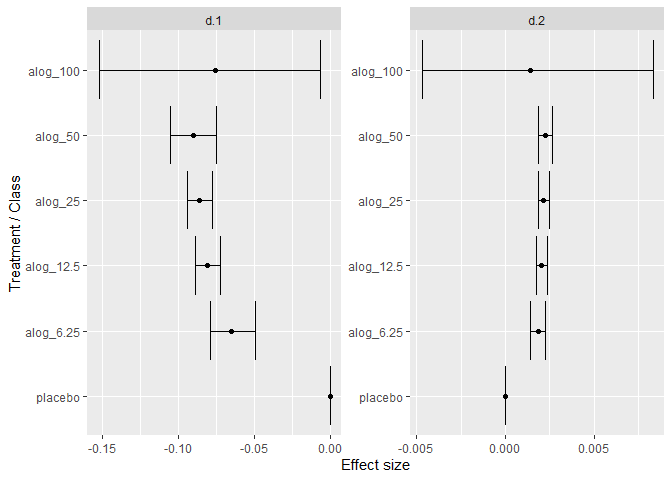### Ranking Rankings can be calculated for different time-course parameters from MBNMA models by using rank() on an "mbnma" object. Any parameter monitored in an MBNMA model that varies by treatment/class can be ranked. A vector of these is assigned to params. direction indicates whether negative scores should be ranked as “better” (-1) or “worse” (1) In addition, it is possible to rank the Area Under the Curve (AUC) for a particular treatment by adding "auc" to the vector of params (included as the default). This will calculate the area under the predicted response over time, and will therefore be a function of all the time-course parameters in the model simultaneously. However, it will be dependent on the range of times chosen to integrate over (int.range), and a different choice of time-frame may lead to different treatment rankings. "auc" can also not currently be calculated from MBNMA models with more complex time-course functions (piecewise, fractional polynomials), nor with MBNMA models that use class effects. # Run a piecewise linear time-course MBNMA with a knot at 1 week mbnma <- mb.piecelinear(network.pain, slope.1=list(pool="rel", method="common"), slope.2=list(pool="rel", method="common"), knot=list(pool="const", method=1)) # Rank results based on AUC (calculated 0-10 weeks), more negative slopes considered to be "better" ranks <- rank(mbnma, params=c("auc", "d.slope.2"), int.range=c(0,10), direction=-1, n.iter=1000) print(ranks) #> ======================================== #> Treatment rankings #> ======================================== #> #> #### d.slope.2 #### #> Treatment Median rank (95% CrI) #> Pl_0 9 (5, 13) #> Ce_100 18 (7, 26) #> Ce_200 17 (12, 22) #> Ce_400 19 (8, 26) #> Du_90 4 (1, 29) #> Et_10 27 (4, 29) #> Et_30 15 (6, 23) #> Et_5 2 (1, 23) #> Et_60 25 (14, 29) #> Et_90 22 (2, 29) #> Lu_100 13 (6, 21) #> Lu_200 14 (8, 22) #> Lu_400 15 (8, 23) #> Lu_NA 3 (1, 28) #> Na_1000 22 (16, 26) #> Na_1500 20 (11, 26) #> Na_250 25 (3, 29) #> Na_750 23 (13, 28) #> Ox_44 4 (1, 26) #> Ro_12 24 (3, 29) #> Ro_125 7 (1, 29) #> Ro_25 26 (13, 29) #> Tr_100 6 (2, 13) #> Tr_200 7 (3, 16) #> Tr_300 10 (4, 20) #> Tr_400 6 (2, 18) #> Va_10 23 (8, 28) #> Va_20 16 (4, 27) #> Va_5 12 (3, 25) #> #> #### auc #### #> Treatment Median rank (95% CrI) #> Pl_0 27 (25, 28) #> Ce_100 21 (16, 23) #> Ce_200 12 (10, 15) #> Ce_400 10 (6.98, 16) #> Du_90 24 (2, 29) #> Et_10 26 (23, 29) #> Et_30 6 (4, 9) #> Et_5 23 (20, 27) #> Et_60 2 (2, 3) #> Et_90 3 (2, 4) #> Lu_100 15 (10, 19) #> Lu_200 16 (12, 20) #> Lu_400 10 (6.98, 13) #> Lu_NA 23 (3, 29) #> Na_1000 5 (4, 7) #> Na_1500 7 (4, 10) #> Na_250 29 (26, 29) #> Na_750 14 (9, 19) #> Ox_44 8 (3.98, 21) #> Ro_12 17 (9, 22) #> Ro_125 1 (1, 1) #> Ro_25 7 (4, 11) #> Tr_100 25 (23, 27) #> Tr_200 24 (22, 26) #> Tr_300 20 (16, 22) #> Tr_400 19 (13, 22) #> Va_10 18 (11, 22) #> Va_20 11 (6, 18) #> Va_5 18 (11, 22) The output is an object of class("mb.rank"), containing a list for each ranked parameter in params, which consists of a summary table of rankings and raw information on treatment ranking and probabilities. The summary median ranks with 95% credible intervals can be simply displayed using print(). Histograms for ranking results can also be plotted using the plot() method, which takes the raw MCMC ranking results given in rank.matrix and plots the number of MCMC iterations the parameter value for each treatment was ranked a particular position. # Ranking histograms for AUC plot(ranks, params = "auc")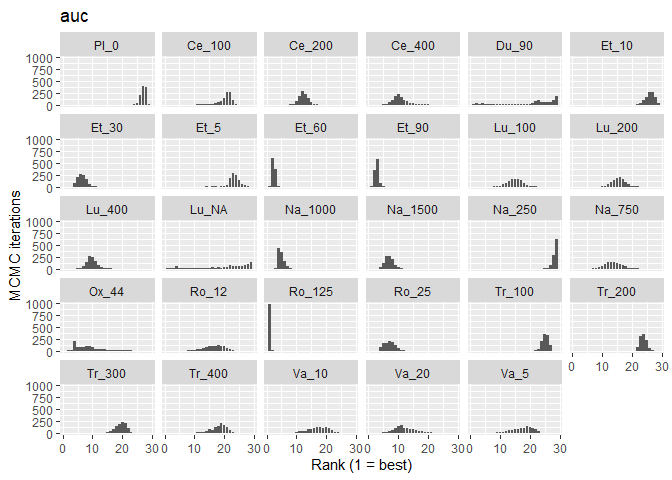### Prediction After performing an MBNMA, responses can be predicted from the parameter estimates using predict() on an "mbnma" object. A number of important parameters need to be identified for this. Additionally, it is also necessary to specify the time points for which to predict responses (times), given as a vector of positive numbers. For further information the help file can be accessed using ?predict.mbnma. The first parameter is E0, which defines what value(s) to use for E0 (time = 0) prediction. A single numeric value can be given for this to indicate a deterministic value, or a distribution can be given as a character to indicate a stochastic value. This should take the form of an R random number generated (RNG) distribution (e.g. "rnorm(n, 7, 0.2)". E0 could be identified for the population of interest from external data (e.g. observational/registry). The second are the values to use for the network reference treatment response. This is only necessary when predicting responses from an MBNMA that pools relative effects for any time-course parameters (pool="rel"), as in this case the network reference treatment response is treated as a nuisance parameter in the modelling. The network reference treatment response is given as ref.resp and can either be estimated from a separate dataset that contains a series of single-arm studies of the network reference treatment, measured at multiple follow-up times. To do this ref.resp is assigned a data frame of studies to synthesise. This could be a series of observational studies that closely match the population of interest, or it could be a selection of data corresponding to the network reference treatment from the same dataset of RCTs used for MBNMA. mbnma <- mb.emax(network.pain, emax=list(pool="rel", method="common"), et50=list(pool="const", method="common")) # Generate a dataset that is made up only of network reference treatment responses over time (in this case placebo) placebo.data <- network.pain$data.ab[network.pain$data.ab$treatname=="Placebo_0",]

# Predict responses for a selection of treatments using a deterministic E0 and placebo.data to estimate the network reference treatment effect
predict.data <- predict(mbnma, treats=c("Pl_0", "Ce_200", "Du_90", "Et_60",
"Lu_400", "Na_1000", "Ox_44", "Ro_25",
"Tr_300", "Va_20"),
times=c(0:15), E0=10,
ref.resp=placebo.data)
# Summary of posterior median predictions
summary(predict.data)
#>       time      Pl_0    Ce_200     Du_90     Et_60    Lu_400   Na_1000
#>  [1,]    0 10.000000 10.000000 10.000000 10.000000 10.000000 10.000000
#>  [2,]    1  8.938316  8.388802  8.559484  7.828007  8.310932  8.162998
#>  [3,]    2  8.719879  8.057289  8.263104  7.381091  7.963392  7.785019
#>  [4,]    3  8.625585  7.914182  8.135164  7.188164  7.813365  7.621853
#>  [5,]    4  8.573020  7.834405  8.063842  7.080614  7.729730  7.530893
#>  [6,]    5  8.539502  7.783534  8.018363  7.012034  7.676400  7.472892
#>  [7,]    6  8.516266  7.748269  7.986836  6.964492  7.639430  7.432684
#>  [8,]    7  8.499210  7.722384  7.963694  6.929595  7.612293  7.403170
#>  [9,]    8  8.486158  7.702575  7.945985  6.902889  7.591526  7.380585
#> [10,]    9  8.475849  7.686928  7.931996  6.881795  7.575122  7.362744
#> [11,]   10  8.467499  7.674255  7.920667  6.864710  7.561837  7.348295
#> [12,]   11  8.460599  7.663783  7.911304  6.850592  7.550858  7.336355
#> [13,]   12  8.454801  7.654983  7.903437  6.838729  7.541633  7.326322
#> [14,]   13  8.449861  7.647486  7.896734  6.828621  7.533773  7.317773
#> [15,]   14  8.445601  7.641021  7.890955  6.819905  7.526995  7.310402
#> [16,]   15  8.441890  7.635389  7.885920  6.812313  7.521091  7.303981
#>           Ox_44     Ro_25    Tr_300     Va_20
#>  [1,] 10.000000 10.000000 10.000000 10.000000
#>  [2,]  8.183738  8.168089  8.487670  8.337661
#>  [3,]  7.810012  7.791163  8.176498  7.995633
#>  [4,]  7.648680  7.628451  8.042171  7.847987
#>  [5,]  7.558743  7.537745  7.967289  7.765680
#>  [6,]  7.501394  7.479906  7.919540  7.713197
#>  [7,]  7.461637  7.439810  7.886438  7.676813
#>  [8,]  7.432455  7.410378  7.862141  7.650107
#>  [9,]  7.410123  7.387856  7.843547  7.629670
#> [10,]  7.392483  7.370065  7.828860  7.613526
#> [11,]  7.378196  7.355656  7.816965  7.600452
#> [12,]  7.366390  7.343749  7.807135  7.589648
#> [13,]  7.356470  7.333745  7.798876  7.580569
#> [14,]  7.348017  7.325220  7.791838  7.572834
#> [15,]  7.340728  7.317869  7.785770  7.566164
#> [16,]  7.334379  7.311466  7.780483  7.560353

Alternatively, values for each time-course parameter modelled using relative effects can be provided by the user as a list, with a separate named element for each time-course parameter. Each element can take either a single numeric value (deterministic), or a RNG distribution (stochastic).

# Define stochastic values for network reference treatment effect on Emax
ref.data <- list("emax"="rnorm(n, -2, 0.15)")

# Predict responses for treatments 1-9 using a stochastic E0 and ref.resp to estimate the network reference treatment effect
predict.resp <- predict(mbnma, treats=c("Pl_0", "Ce_200", "Du_90", "Et_60",
"Lu_400", "Na_1000", "Ox_44", "Ro_25",
"Tr_300", "Va_20"),
times=c(0:15), E0="rnorm(n, 9, 0.05)",
ref.resp=ref.data)

An object of class "mb.predict" is returned, which is a list of summary tables and MCMC prediction matrices for each treatment, in addition to the original mbnma object. The summary() method can be used to print mean posterior predictions at each time point for each treatment.

Predicted responses can also be plotted using the plot() method on an object of class("mb.predict"). Within the default arguments, the median predicted network reference treatment response is overlaid on the predicted response for each treatment. Setting overlay.ref = FALSE prevents this and causes the network reference treatment predicted response to be plotted as a separate panel. Shaded counts of observations in the original dataset at each predicted time point can be plotted over the 95% CrI for each treatment by setting disp.obs = TRUE.

plot(predict.resp, overlay.ref=TRUE, disp.obs=TRUE)
#> Reference treatment in plots is Pl_0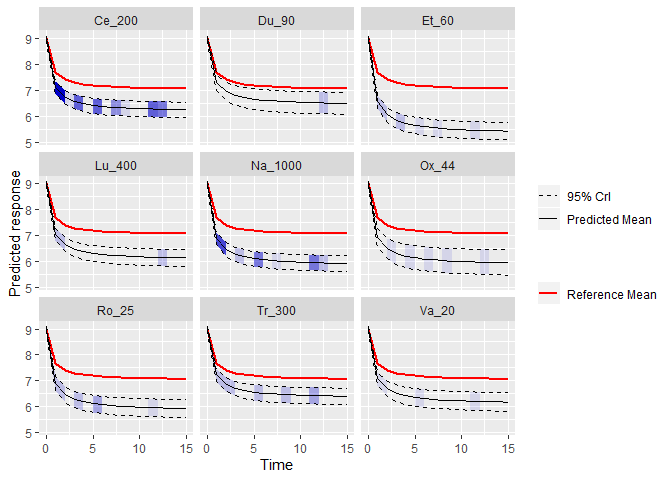This can be used to identify any extrapolation/interpretation of the time-course that might be ocuring for a particular treatment.

To illustrate a situation in which this could be very informative, we can look at predicted responses for a quadratic time-course function fitted to the Obesity dataset:

# Fit a quadratic time-course MBNMA to the Obesity dataset
beta.1 = list(pool="rel", method="common"),
beta.2 = list(pool="rel", method="common")
)

# Define stochastic values centred at zero for network reference treatment
ref.data <- list(beta.1="rnorm(n, 0, 0.05)", beta.2="rnorm(n, 0, 0.0001)")

# Predict responses over the
predict.resp <- predict(mbnma, times=c(0:50), E0="rnorm(n, 120,1)", treats = c(1,4,15),
ref.resp=ref.data)
#> Priors required for: beta.1, beta.2
#> Success: Elements in prior match consistency time-course treatment effect parameters

# Plot predictions
plot(predict.resp, disp.obs = TRUE)
#> Reference treatment in plots is plac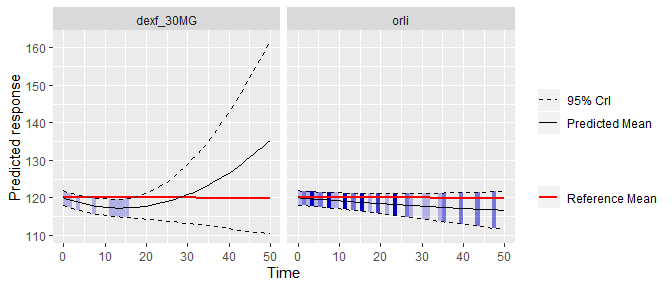As you can see, within the limits of the observed data the predicted responses appear reasonable. However, extrapolation beyond this in treatment 4 leads to some rather strange results, suggesting a huge increase in body weight after 50 weeks of treatment. On the other hand, the predicted response at 50 weeks follow-up in treatment 15 is within the limits of the observed data and so are likely to be more justifiable.

## Consistency Testing

When performing an MBNMA by pooling relative treatment effects (pool="rel"), the modelling approach assumes consistency between direct and indirect evidence within a network. This is an incredibly useful assumption as it allows us to improve precision on existing direct estimates, or to estimate relative effects between treatments that have not been compared in head-to-head trials, by making use of indirect evidence.

However, if this assumption does not hold it is extremely problematic for inference, so it is important to be able to test it. A number of different approaches exist to allow for this in standard Network Meta-Analysis (Dias et al. 2013). Two of these have been implemented within MBNMAtime. It is important to note that in some model specifications there is likely to be sharing of model parameters (e.g. heterogeneity parameters, correlation coefficients) across networks which will lead to more conservative tests for consistency, and may lead to an inflated type II error.

Consistency is also likely to differ depending on the model used. Failing to appropriately model the time-course function may in fact induce inconsistency in the data. “Lumping” together different time points from studies in standard NMA is known to be a potential cause of inconsistency, which is one of the reasons why accounting for time-course using MBNMA is important (Pedder et al. 2019). When performing MBNMA, this is why it is important to first try to identify the best model possible in terms of time-course and common/random effects, and then to test for consistency within that model, rather than testing for consistency in models that are known not be be a good fit to the data.

Consistency testing can only be performed in networks in which closed loops of treatment comparisons exist that are drawn from independent sources of evidence. In networks which do not have any such loops of evidence, consistency cannot be formally tested (though it may still be present). The mb.nodesplit.comparisons() function identifies loops of evidence that conform to this property, and identifies a treatment comparison within that loop for which direct and indirect evidence can be compared using node-splitting (see below).

# Loops of evidence within the alogliptin dataset
splits.alog <- mb.nodesplit.comparisons(network.alog)
print(splits.alog)
#>   t1 t2    path
#> 8  3  4 3->1->4
#> 7  2  5 2->1->5
#> 6  2  4 2->1->4
#> 5  2  3 2->1->3

### Unrelated Mean Effects (UME) model

To check for consistency using UME we fit a model that does not assume consistency relationships, and that only models the direct relative effects between each arm in a study and the study reference treatment. If the consistency assumption holds true then the results from the UME model and the MBNMA will be very similar. However, if there is a discrepancy between direct and indirect evidence in the network, then the consistency assumption may not be valid, and the UME results are likely differ in several ways:

• The UME model may provide a better fit to the data, as measured by deviance or residual deviance
• The between-study SD for different parameters may be lower in the UME model
• Individual relative effects may differ in magnitude or (more severely) in direction for different treatment comparisons between UME and MBNMA models

UME can be fitted to any time-course parameter which has been modelled using relative effects (pool="rel"). UME can be specified for each time-course parameter in separate analyses, or can be modelled all at once in a single analysis.

# Fit a piecewise linear MBNMA with fixed relative effects on slope.1 and slope.2
mbnma <- mb.piecelinear(network.pain,
slope.1=list(pool="rel", method="common"),
slope.2=list(pool="rel", method="common"),
knot=list(pool="const", method=0.5),
pd="pd.kl")

# Fit a UME model on both slope parameters simultaneously in a piecewise linear MBNMA
ume <- mb.piecelinear(network.pain,
slope.1=list(pool="rel", method="common"),
slope.2=list(pool="rel", method="common"),
knot=list(pool="const", method=0.5),
UME=TRUE, pd="pd.kl")

# Fit a UME model on slope.1 only in a piecewise linear MBNMA
ume.slope.1 <- mb.piecelinear(network.pain,
slope.1=list(pool="rel", method="common"),
slope.2=list(pool="rel", method="common"),
knot=list(pool="const", method=0.5),
UME="slope.1", pd="pd.kl")

# Fit a UME model on slope.2 only in a piecewise linear MBNMA
ume.slope.2 <- mb.piecelinear(network.pain,
slope.1=list(pool="rel", method="common"),
slope.2=list(pool="rel", method="common"),
knot=list(pool="const", method=0.5),
UME="slope.2", pd="pd.kl")
#>  "Deviance for mbnma: -105.36"
#>  "Deviance for ume on slope.1 and slope.2: -115.84"
#>  "Deviance for ume on slope.1: -118.22"
#>  "Deviance for ume on slope.2: -116.18"

By comparing the deviance (or residual deviance) of models with UME fitted on different time-course parameters and the MBNMA model, we can see that there is some reduction in deviance in the different UME models. Given that deviance is lowest when UME is modelled only on slope.1 this is suggestive of inconsistency between direct and indirect evidence on slope.1, but perhaps also on slope.2 given that modelling UME on this also leads to a reduction in deviance.

# Run an Emax MBNMA with random relative effects on emax
mbnma <- mb.emax(network.gout,
emax=list(pool="rel", method="random"),
et50=list(pool="const", method="common"),
n.iter=10000, n.thin=10,
intercept=FALSE)

# Fit a UME model on Emax parameters
ume <- mb.emax(network.gout,
emax=list(pool="rel", method="random"),
et50=list(pool="const", method="common"),
n.iter=10000, n.thin=10,
intercept=FALSE, UME=TRUE)
#>  "Deviance for mbnma: 2127.18"
#>  "Deviance for UME: 2127.18"

In the gout dataset, the deviances for MBNMA and UME models are very similar when modelling an Emax time-course, suggesting that the consistency assumption is likely to be reasonable.

As these model random relative treatment effects, we can also compare the between-study SDs. The SD for the UME model is slightly higher, suggesting that assuming consistency in the MBNMA model is not leading to higher between-study SD. This is further evidence to support the consistency assumption.

#>  "SD for mbnma: 0.76"
#>  "SD for UME: 0.89"

Direct estimates from UME and MBNMA models can also be compared to examine in greater detail how inconsistency may be affecting results. However, it is important to note that whilst a discrepancy between UME and MBNMA results may be seen for a particular relative effect, the inconsistency is not exclusively applicable to that particular treatment comparison and may originate from other comparisons in the network. This is why consistency checking is so important, as a violation of the consistency assumption raises concerns about estimates for all treatments within the network.

### Node-splitting

Another approach for consistency checking is node-splitting. This splits contributions for a particular treatment comparison into direct and indirect evidence, and the two can then be compared to test their similarity. mb.nodesplit() takes similar arguments to mb.run() that define the underlying MBNMA model in which to test for consistency, and returns an object of class("mb.nodesplit"). Currently node-splitting does not work with any of the wrapper function for mb.run(). There are two additional arguments required:

comparisons indicates on which treatment comparisons to perform a node-split. The default value for this is to automatically identify these using mb.nodesplit.comparisons().

nodesplit.parameters indicates on which time-course parameters to perform a node-split. This can only take time-course parameters that have been assigned relative effects in the model (pool="rel"). Alternatively the default "all" can be used to split on all available time-course parameters in the model that have been pooled using relative effects.

As up to two models will need to be run for each treatment comparison to split, this function can take some time to run.

# Nodesplit using an Emax MBNMA
nodesplit <- mb.nodesplit(network.pain, fun="emax",
beta.1=list(pool="rel", method="random"),
beta.2=list(pool="const", method="common"),
nodesplit.parameters="all")
print(nodesplit)
#> ========================================
#> Node-splitting analysis of inconsistency
#> ========================================
#>
#>
#> #### beta.1 ####
#>
#> comparison   p.value         Median (95% CrI)
#> d.3.22       0.8493
#> -> direct                0.379 (-0.441, 1.19)
#> -> indirect              0.454 (-0.326, 1.27)
#> d.3.15       0.5209
#> -> direct                0.224 (-0.213, 0.664)
#> -> indirect              0.367 (-0.39, 1.13)

Performing the print() method on an object of class("mb.nodesplit") prints a summary of the node-split results to the console, whilst the summary() method will return a data frame of posterior summaries for direct and indirect estimates for each split treatment comparison and each time-course parameter.

The node-split object itself is a list with results for each time-course parameter, for each treatment comparison that has been split. There is a lot of information within the results, but the most useful (and easily interpretable) elements are:

• p.values the Bayesian p-value for the posterior overlap between direct and indirect estimates
• quantiles the median and 95%CrI of the posterior distributions for direct and indirect evidence, and for the difference between them, as well as the pooled (MBNMA) result.
• forest.plot a forest plot that shows the median and 95% CrI for direct and indirect estimates
• density.plot a plot that shows the posterior distributions for direct and indirect estimates

It is possible to generate different plots of each node-split comparison using plot():

# Plot forest plots of direct and indirect results for each node-split comparison
plot(nodesplit, plot.type="forest")

# Plot posterior densities of direct and indirect results for each node-split comparisons
plot(nodesplit, plot.type="density")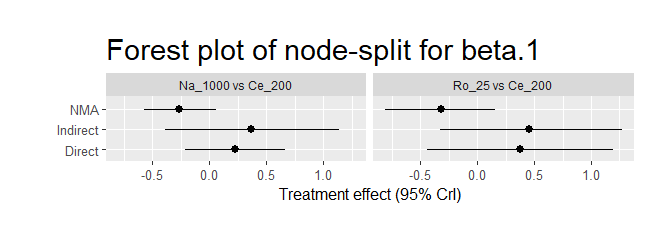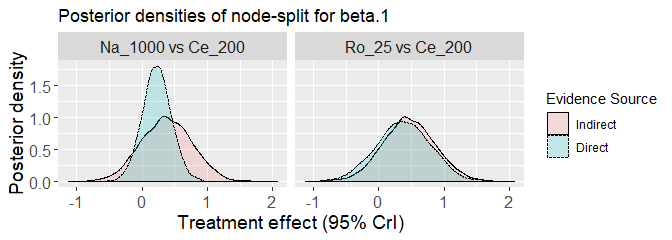As a further example, if we use a different time-course function (piecewise linear - as in the section on UME models) that is a less good fit for the data, and perform a node-split on both beta.1 and beta.2 time-course parameters, we find that there seems to be a strong discrepancy between direct and indirect estimates for beta.1 and a smaller, yet still potentially important discrepancy for beta.2. This is in agreement with the results from the UME models, that were suggestive of inconsistency in this MBNMA model. This is strong evidence to reject the consistency assumption, and to either (as in this case) try to identify a better fitting model, or to re-examine the dataset to try to explain why this might be the case.

This highlights the importance of testing for consistency after identifying an appropriate time-course and common/random effects model.

# Nodesplit on beta.1 and beta.2 using a piecewise linear MBNM
ns.plin <- mb.nodesplit(network.pain, fun="piecelinear",
beta.1=list(pool="rel", method="common"),
beta.2=list(pool="rel", method="common"),
beta.3=list(pool="const", method=0.5),
nodesplit.parameters="all")
print(ns.plin)
#> ========================================
#> Node-splitting analysis of inconsistency
#> ========================================
#>
#>
#> #### beta.1 ####
#>
#> comparison   p.value         Median (95% CrI)
#> d.3.22       0.3599
#> -> direct                0.242 (-1.98, 2.41)
#> -> indirect              0.521 (-0.19, 1.26)
#> d.3.15       0.008919
#> -> direct                -0.198 (-0.656, 0.251)
#> -> indirect              1.17 (0.578, 1.73)
#>
#>
#> #### beta.2 ####
#>
#> comparison   p.value         Median (95% CrI)
#> d.3.22       0.4359
#> -> direct                0.00669 (-0.114, 0.125)
#> -> indirect              0.0123 (-0.0362, 0.0616)
#> d.3.15       0.1013
#> -> direct                0.0115 (-0.0162, 0.0387)
#> -> indirect              -0.0383 (-0.0792, 0.00345)

plot(ns.plin, plot.type="forest")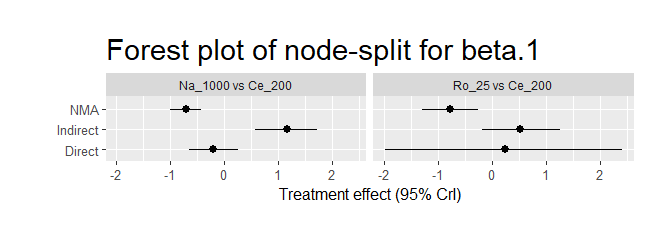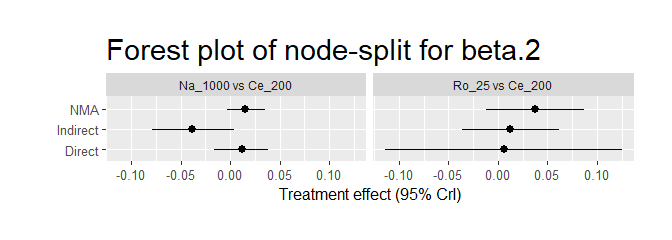## Conclusions

MBNMAtime provides a complete set of functions that allow for meta-analysis of longitudinal time-course data and plotting of a number of informative graphics. Functions are also provided for prediction, and for assessing consistency when modelling using relative effects. By accounting for time-course in meta-analysis this can help to explain heterogeneity/inconsistency that may arise when using conventional NMA.

The package allows for modelling of either relative or arm-based absolute effects interchangeably on different time-course parameters within the same analysis, whilst allows users wishing to perform MBNMA/MBMA a great deal of flexibility in their modelling decisions, whilst providing a straightforward syntax with which to define these models.